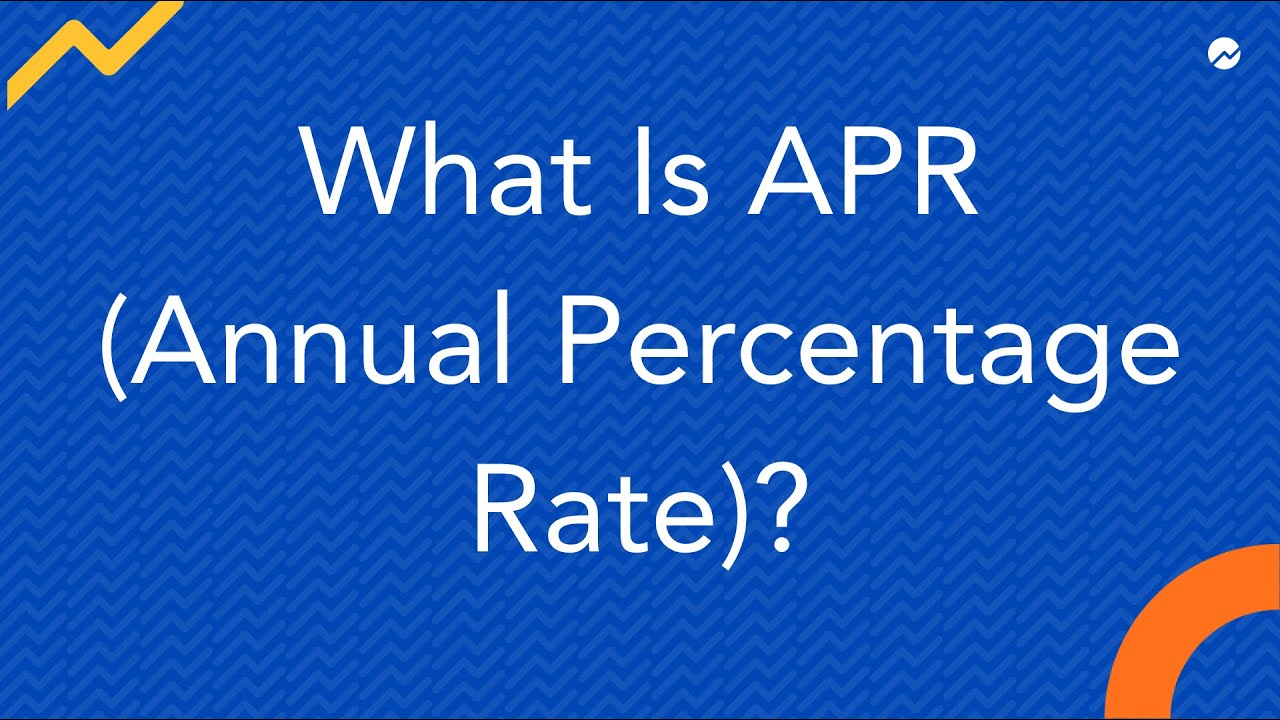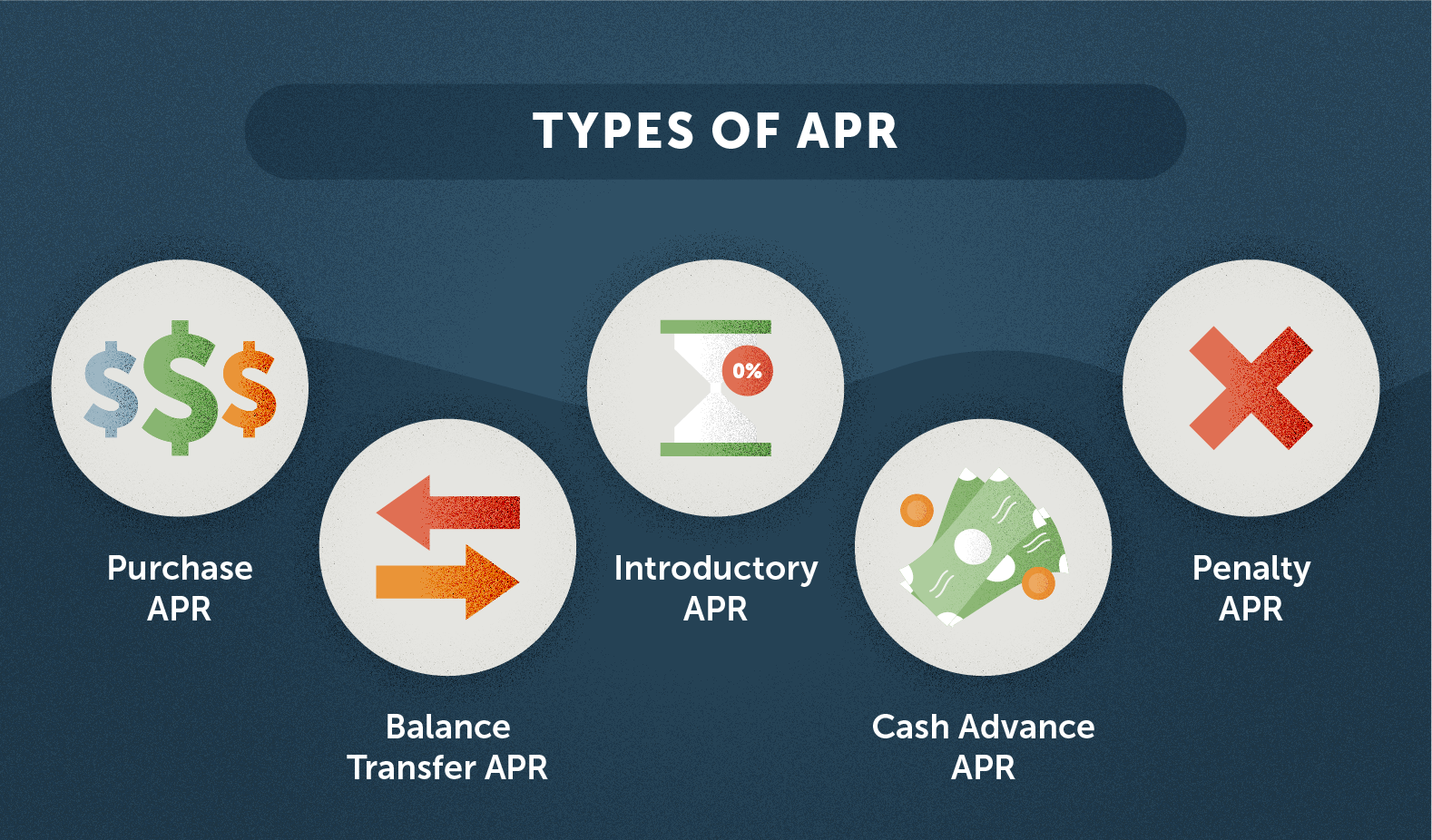# How to calculate APR (Annual Percentage Rate) on a Loan

Shopping for a car can feel overwhelming between choosing a brand, model, and options. And there you are not done there. Car loan shopping is just as important—and knowing how to calculate your APR for a vehicle loan can help you determine if a loan is right for you.

## What is APR?The annual percentage rate (APR) is the annual rate of interest charged to borrowers and paid to investors. APR is a percentage that represents the actual yearly cost of funds over the life of a loan or the income earned on an investment. This includes any fees or additional costs associated with the transaction but does not account for compounding. The APR gives consumers a single number that they can easily compare to rates from other lenders.

## How to Calculate APR?The estimated APR on a car loan can be calculated using computer spreadsheet software. You’ll need the following information to go that route:

• Loan amount — The total amount you intend to finance, usually the vehicle’s purchase price minus any down payment or trade-in (a down payment or trade-in will lower the amount you need to finance, which can reduce your monthly payment)
• Loan term — The length of time you have your car loan.
• The interest rate on the loan (this is an estimated rate until you formally apply) Certain fees, such as origination fees,

## Method of Calculating APR### 1. Determine your monthly estimated payment.

You can skip this step if you already know your estimated monthly loan payment. If you don’t have one, you can easily calculate your monthly car payment on a spreadsheet by entering the formula below into a cell.

=PMT (interest rate as a decimal/12, loan term in months, loan amount with fees)

As a result, your monthly payment is estimated. It will be a negative number, but don’t be concerned. You did not make an error. Save this number for calculating your APR.

#### For instance,

Assume you want to finance 10,00,000 INR (9,50,000 plus a 50,000 INR loan application fee) with a loan term of 60 months and a 4% interest rate. Here’s what your formula would look like if those numbers were entered.

=PMT(.04/12, 60, 1000000)

### 2. Determine your estimated APR.

To calculate your loan’s APR using a spreadsheet, enter the formula below into a cell. This formula assumes that your monthly payment was calculated in step 1 or that it includes fees in any case. If you did not calculate your monthly payment in step 1 or are unsure whether the monthly payment, you’re using includes fees, keep in mind that this formula may not be the most accurate way to calculate your estimated APR.

=RATE(months in loan term, estimated monthly payment, loan value minus fees)*12

Here’s what you’d enter into the cell for this loan example using the monthly payment you calculated (-18,416).

RATE(60,-18416,950000)*12

Entering the formula above would calculate your estimated APR at approximately 6.1%.

## What is a good APR for a car loan?On 48- and 60-month car loans, commercial banks charged an average APR of 4.98 percent. However, keep in mind that interest rates differ by lender, and a variety of other factors can influence the APR you’re offered.